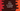# C++ program to check if a number is perfect or not# Introduction :

A perfect number is a number that is equal to the sum of its positive divisors excluding the number itself. For example, 28 is a perfect number because the sum of its divisors is 1 + 2 + 4 + 7 + 14 = 28 _. Similarly, _6 is also a perfect number because 1 + 2 + 3 = 6.

We can write one C++ program to check if a number is perfect or not. For that, we need to find out the sum of all divisors of the number. In this post, I will show you how to write one C++ program to find out if a number is perfect or not. The program will take one number as input from the user and print out if it is a perfect number or not.

## C++ program :

``````#include <iostream>
using namespace std;

bool checkPerfect(int no)
{
// 3
int i = 0;
int sum = 0;

// 4
while (i++ < no)
{
// 5
if (no % i == 0 && i < no)
{
sum += i;
}
}

return sum == no;
}

int main()
{
int n;

// 1
cout << "Enter a number : " << endl; cin >> n;

// 2
if (checkPerfect(n))
{
cout << "It is a perfect number" << endl;
}
else
{
cout << "It is not a perfect number" << endl;
}

return 0;
}``````

### Explanation :

The commented numbers in the above program denote the step numbers below :

1. Ask the user to enter a number. Read that number and store it in variable n.
2. Check if the number is perfect or not using checkPerfect method. It returns one boolean value. Print the message accordingly.
3. Create two integer variables i and sum. sum is for holding the total sum.
4. Run one while loop and check for all numbers from 1 to no.
5. If the current number can divide no, add it to the variable sum. Finally check if the sum is equal to the provided number or not. If yes, return true, else return false.

### Sample Output :

``````Enter a number :
28
It is a perfect number

Enter a number :
21
It is not a perfect number

Enter a number :
6
It is a perfect number``````